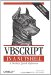# Section A.6. Date and Time

### A 6 Date and Time

 CDate Fujnction Converts a value to a date Date Function Returns the current system date DateAdd Function Returns the result of a data/time addition or subtraction calculation DateDiff Function Returns the difference between two dates DatePart Function Returns the part of the date requested DateSerial Function Returns a date from an expression containing month, day, and year DateValue Function Returns a date from a representation of a date Day Function Returns a number representing the day of the month FormatDateTime Function Returns a string variant formatted using the date settings for the current locale Hour Function Returns a number representing the hour of the day Minute Function Returns a number representing the minute of the hour Month Function Returns a number representing the month of the year MonthName Function Returns the name of the month for a given date Now Function Returns the current system time Second Function Returns a number representing the second of the minute Time Property Returns or sets the current system time Timer Property Returns the number of seconds elapsed since midnight TimeSerial Function Returns a representation of a given hour, minute, and second TimeValue Function Returns a time value from a string representation of a time Weekday Function Returns a number representing the day of the week WeekdayName Function Returns a string indicating the day of the week Year Function Returns a number representing the year in a date expressionVBScript in a Nutshell, 2nd Edition
ISBN: 0596004885
EAN: 2147483647
Year: 2003
Pages: 335